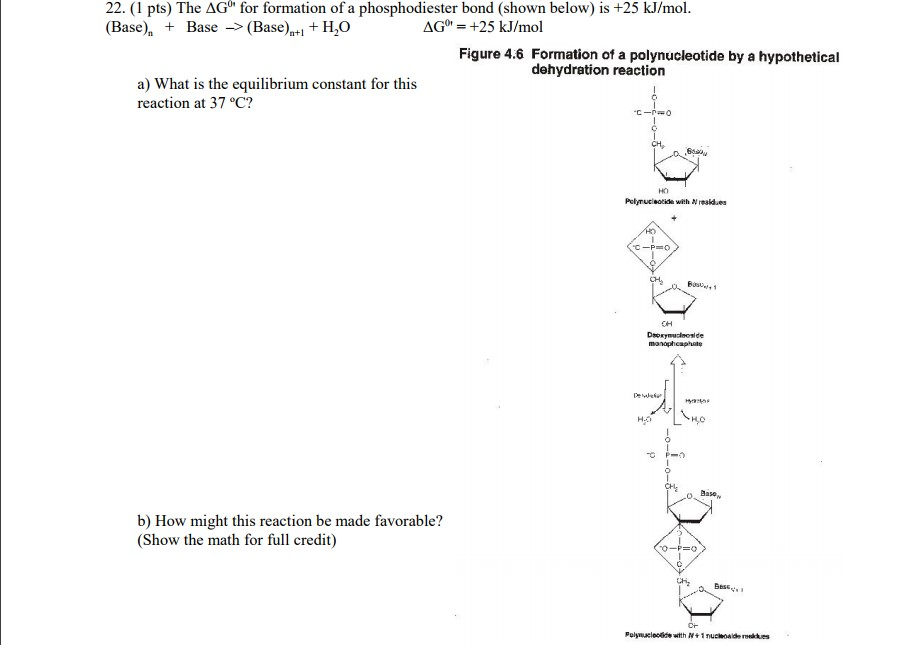# Question & Answer: The Delta G^0 for formation of a phosphodiester bond (shown below) is +25 kJ/mol. (Base)_n…..The Delta G^0 for formation of a phosphodiester bond (shown below) is +25 kJ/mol. (Base)_n + Base rightarrow (Base)_n + 1 + H_2O Delta G^0 = +25 kJ/mol a) What is the equilibrium constant for this reaction at 37 degree C? b) How might this reaction be made favorable? (Show the math for full credit)

Given

Don't use plagiarized sources. Get Your Custom Essay on
Question & Answer: The Delta G^0 for formation of a phosphodiester bond (shown below) is +25 kJ/mol. (Base)_n…..
GET AN ESSAY WRITTEN FOR YOU FROM AS LOW AS \$13/PAGE

G of the reaction = +25KJ/mole = +25 * 103 J/mole

T = 370C + 273k = 310 K

we know

G = -RTlnKeq

+25 * 103 J/mole = – 8.314 J/K.mole * 310K * lnKeq

on solving equilibrium constant Keq = 6.2 * 10-5

b.

here equilibrium constant is less than 1. So it is reactant favourable. i.e, backward reaction occurs mostly.